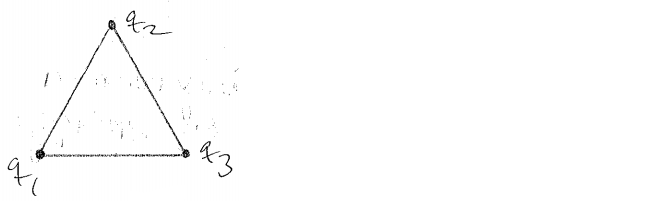# Problem: Three equal point charges, each with charge 1.35 μC, are placed at the vertices of an equilateral triangle whose sides are of length 0.250 m. What is the electric potential energy U of the system? (Take as zero the potential energy of the three charges when they are infinitely far apart.) Use ϵ0 = 8.85 x 10-12 C2/Nm2 for the permittivity of free space.

###### FREE Expert Solution
99% (406 ratings)
###### Problem Details

Three equal point charges, each with charge 1.35 μC, are placed at the vertices of an equilateral triangle whose sides are of length 0.250 m. What is the electric potential energy U of the system? (Take as zero the potential energy of the three charges when they are infinitely far apart.) Use ϵ0 = 8.85 x 10-12 C2/Nm2 for the permittivity of free space.